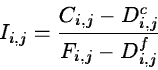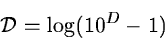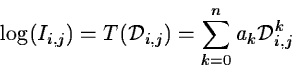Next: Geometric Corrections Up: Raw to Calibrated Data Previous: Artifacts

Response Calibration

The raw data values from the detector system have to be transformed into relative intensities which then can later be adjusted to an absolute scale by comparison with standard objects. The majority of modern detectors (e.g. CCD, diode-array or image tube) have a nearly linear response while photographic emulsions are strongly non-linear. Even for the `linear' detectors, a number of corrections must be included in the intensity calibration. Some of these can be derived theoretically such as dead-time corrections for photon counting devices or saturation effects for electronographic emulsions while other non-linear effects are determined empirically. Systems which are assumed to be linear need only be corrected for a possible dark count and bias in addition to the relative sensitivity variation over the detector. The correction frames are determined from a set of test exposures from which artifacts are removed first as described in Section 2.2.1. A raw frame Ci,j is then transformed to relative intensities Ii,j by(2.10)

where Di,j is the appropriate dark counts including bias and Fi,j is a normalized flat field exposure.

A mathematical function is used to transform data from detectors with non-linear response to a more linear domain. For photographic emulsions Baker (1925) found the formula(2.11)

which makes the lower part of the characteristic curve almost linear. In Equation 2.11, D is the photographic density above fog. These values can then, by means of least squares methods, be fitted with a power series(2.12)

where n for most applications is smaller than 7. The characteristic curves are shown in Figure 2.4 both using normal and Backer densities. The coefficients ak depend not only on the emulsion type but also on the spectral range. For spectral plates this leads to a positional variation of the terms ak.The main problem with non-linear detectors is not so much to determine the response curve as the modification of the noise distribution. Thus, the gaussian grain noise on emulsions becomes skewed through the intensity calibration. Special care must be taken in the further processing to avoid systematic error due to non-gaussian noise (e.g. the average of a region will be biased). One possible way to make the distribution more gaussian again is to apply a median filter because it is less affected by the transformation.Next: Geometric Corrections Up: Raw to Calibrated Data Previous: Artifacts
Petra Nass
1999-06-15